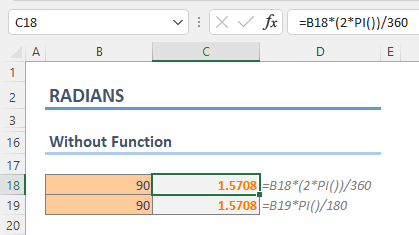In Excel, The RADIANS is a Math & Trigonometry function that converts degrees into radians. In this guide, we’re going to show you how to use the RADIANS function and go over some tips and error handling methods.

• All versions

## Arguments

 angle A degree value that you want to convert.

## Examples

A radian is a unit of measurement of angles. One radian is defined as the angle subtended from the center of a circle which intercepts an arc equal in length to the radius of the circle.

The math behind the calculation is simple:Which can be simplified into:You can use PI function to return π value.

All you need to do is to supply the angle degreed you want to convert.